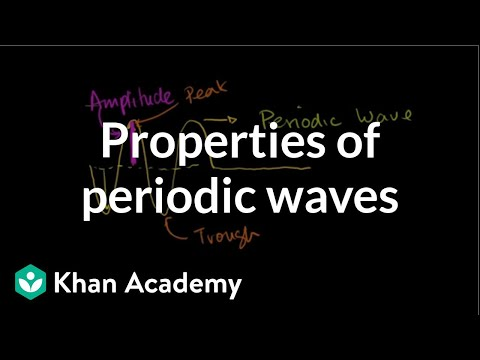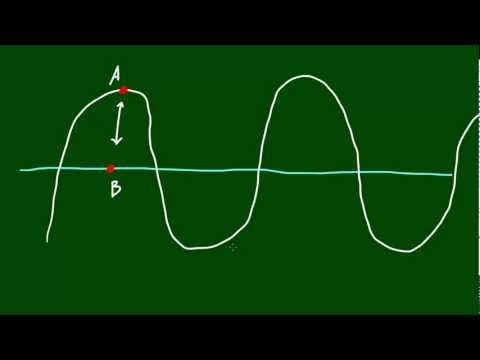## Some Terms Related with Waves

Subject: Science

#### Overview

One crest and one trough constitute one complete wave in a transverse wave. Longitudinal wave travels in the form of compression and rarefaction. This note provide us the basic idea about wave and its types.
##### Some Terms Related with Waves#### Crest and trough

When the displacement of a particle is maximum above from its mean position, the particle is said to be at the crest of the wave and when the displacement of a particle is maximum below the mean position, the particle is said to be at the trough. The transverse wave travels in the form of trough and crest.#### Compression and rarefaction

TheLongitudinal wave travels in the form of compression and rarefaction. In compression, particles of medium get compressed and in rarefaction density is less than normal density. In another way, the region where the medium is compressed is known as a compression and the region where the medium is spread out is known as a rarefaction.#### A complete wave

One crest and one trough constitute one complete wave in a transverse wave. One compression and one rarefaction constitute one complete wave in a longitudinal wave.#### Amplitude (a)

The maximum displacement of a particle from its mean position in a wave is called amplitude of that wave. Its SI unit is ‘m’.#### Frequency (f)

The number of complete waves, set up in a medium in one second is called frequency of the wave. Its SI unit is hertz (Hz).

#### Time period (T)

The time taken by wave to complete one cycle or a complete wave is called time period. Its unit is second.#### Wavelength (λ)

The distance between two consecutive troughs or crests in a transverse wave or the distance between two consecutive compressions or rarefactions in a longitudinal wave is called wavelength. Its SI unit is meter (m).

#### Wave velocity (v)

The velocity with which wave propagates in a medium is called wave velocity. Its SI unit is m/s.

#### Wave equation

The formula showing the relation between v, f and λ is called wave equation. The product of frequency and wavelength is equal to wave velocity.

Wave velocity (v) = frequency (f) × wavelength (λ)

#### Relation between frequency (f) and time period (T)

The number of complete waves, set up in a medium in one second is called frequency of the wave. Its SI unit is hertz (Hz). According to the definition of frequency,
f vibrations are completed in one second.
Or, 1 vibrations is completed in $\frac{1}{f}$ second.
But, time to complete one vibrationis its time period (T)
$\therefore$ T = $\frac{1}{f}$

 time period = $\frac{1}{frequency}$

##### Things to remember
• One crest and one trough constitute one complete wave in a transverse wave.
• Longitudinal wave travels in the form of compression and rarefaction.
• The maximum displacement of a particle from its mean position in a wave is called amplitude
• The number of complete waves, set up in a medium in one second is called frequency
• The velocity with which wave propagates in a medium is called wave velocity
• It includes every relationship which established among the people.
• There can be more than one community in a society. Community smaller than society.
• It is a network of social relationships which cannot see or touched.
• common interests and common objectives are not necessary for society.
##### Videos for Some Terms Related with Waves##### Amplitude, Period, Frequency and Wavelength of Periodic Waves##### Questions and Answers
When the displacement of the particle maximum above its mean position, it is called crest.
The regions in medium where the density of particles of a medium is less are called rarefaction.

One crest and one trough in transverse wave and one compression and one rarefaction in longitudinal wave is called a complete wave.

The number of waves (or cycles) produced by the sources of sound in one second is frequency of sound. It is denoted by f or µ and unit is cycle/sec (hertz).
The distance between two consecutive troughs or crests in a transverse wave or the distance between two consecutive compressions or rarefactions in longitudinal waves is called wavelength. It is denoted by λ and its SI unit is meter (m).
The maximum displacement of a particle from its mean position is called amplitude. It is denoted by 'a'.
The relationship between wavelength (λ) , frequency (f) and velocity of wave (v) is called wave equation. The wave equation is defined as the product of frequency and wavelength and is numerically equal to the wave velocity.
i.e. Wave velocity (V) = Wavelength (λ) x Frequency (f)
V = λ x f
The frequency of boy's voice is least (i.e. 6.5 KHz) than that of girl's (i.e. 8.5KHz). We know that, for the same medium when the speed is constant the wavelength varies inversely with frequency (i.e. λ ∝). So, as the frequency increases, the wavelength decreases. Due to this reason, the wavelength of girl's voice is shorter than that of boys.
The sound wave with high amplitude has more intensity and carries more energy than that of the sound with low amplitude. So, such wave is transmitted to a longer distance.
The process of bending of sound wave while passing from one medium to another is called rarefaction of sound. It follows all the rules of refraction of light.
Solution:
Given,
Speed of sound (v) = 350m/s
Wavelength (λ) = 35m
Frequency of sound (f) = ?
We know,
v = λ x f
or, f =or, f =∴Frequency = 10 Hz

Solution:
Here,
Speed of sound (v) = 340m/s
Wavelength (λ) = 2.4cm = 0.024m
Frequency of sound (f) = ?
We know,
v = λ x f
or, f =or, f =∴Frequency = 14166.67 Hz

Solution:
Here,
Speed of sound (v) = 332m/s
Wavelength (λ) = ?
Frequency of sound (f) = 20Hz
We know,
v = λ x f
or, λ =or, λ =∴Wavelength = 16.6m
Solution:
Here,
Speed of sound (v) = ?
Wavelength (λ) = 0.012m
Frequency of sound (f) = 20KHz = 20 x 1000 = 20000Hz
We know,
v = λ x f
= 20000 x 0.012
= 240m/s
Hence, the speed of the sound is 240 m/s.

Solution:
Here,
Speed of sound (v) = 340m/s
Shortest Wavelength (λ) = ?
Highest Frequency of sound (f) = 20KHz = 20 x 1000 = 20000Hz
We know,
v = λ x f
or, λ =or, λ =∴ λ = 0.017m
Hence, the shortest wavelength is 0.017m
Again similarly,
We have,
Speed of sound (v) = 340m/s
Longest Wavelength (λ) = ?
lowest Frequency of sound (f) = 20Hz
We know,
v = λ x f
or, λ =or, λ =∴ λ = 17m
Hence, the longest wavelength is 17m

Solution:
Here,
Speed of radio wave (v) = 3 x 108 m/s
Wavelength (λ) = ?
Frequency of sound (f) = 103.6MHz = 103.6 x 1000000 = 103600000Hz
We know,
v = λ x f
or, λ =or, λ =∴Wavelength = 0.29m

Solution:
Here,
Total number of complete wave (n) = 20
Time (t) = 4s
Frequency (f) = ?
We have,
f == 20/4 = 5 Hz
Also, the distance between two crests is 3 cm
So, wavelength (λ) = 3/100 = 0.03m
Again,
Wave speed (v) = wavelength (λ) x frequency (f)
= 0.03 x 5
= 0.15m/s

1. Since the velocity of sound is inversely proportional to the square root of density of gas, A has highest density and B has least.
1. We have,
v = λ x f
or, λ ∝ v
i.e. wavelength is directly proportional to the velocity of the sound. Since, velocity of sound is greater in medium B and least in A, the wavelength of the sound will be highest in B and lowest in A.
1. The sharpness of a sound depends on frequency. So, 'A' is baby, 'B' is woman and 'C' is an adult man.
2. Solution: We know,
v = λ x f
or, λ =or, λ ∝Hence, the frequency of an adult man 'C' has minimum so 'C' has greatest wavelength.
3. The frequency of sound produced by A is 900 Hz, so 900 crests and 900 troughs are produced per second.